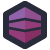Just because someone writes in C, and not some more modern language doesn't mean they're going to be an expert in how to operate on binary values.

Carl W found this bit of C code with a simple goal: count the number of trailing zeroes in a 32-bit integer. At least, that's what the function name tells us. Reading the logic, I'm less certain.

``````u32_t FCN_count_trailing_zeros(u32_t x)
{
u32_t c = 32u; // c will be the number of zero bits on the right
if (x != 0u) // if n == 0, skip and return c = 32
{
x &= (unsigned)-(signed)x;
c = 31u;
if ((x & 0x0000FFFFu) != 0u)
{
c = 15u;
}
if ((x & 0x00FF00FFu) != 0u)
{
c -= 8u;
}
if ((x & 0x0F0F0F0Fu) != 0u)
{
c -= 4u;
}
if ((x & 0x33333333u) != 0u)
{
c -= 2u;
}
if ((x & 0x55555555u) != 0u)
{
c -= 1u;
}
}
return c;
}
``````

I'm a simple person, so I'd be tempted to just do this with a loop and a bit-shift operation. Now, it's probably wrong to say that this programmer doesn't know how to operate on binary values. I think they've got a better grasp on it than most of us, because it's hard to understand what's going on here but this code works, at least after a few quick tests.

We start by assuming that, at most, a 32-bit integer can contain 32 zeros, by setting `c = 32u`. That seems pretty reasonable, at first glance.

Some of it just looks bizarre at first glance, if you aren't used to some of the more unique C idioms: `x &= (unsigned)-(signed)x;`

This converts `x` to a signed value, negates it, then converts it back to an unsigned value. In C, this involves adding the maximum int plus one to the negative value until it's positive. When then and this with the original value. Now, assuming max-int is `0xFFFFFFFF`, this has an interesting effect: it strips numbers to their first significant bit. `256` stays `256`, but `257` becomes `1`- because the first bit has to be set.

Great, now that we've done that, we assume at most `31u` leading zeros (we've discarded zero as a possibility at this point in that conditional). Then we do a series of `&` operations with bitmasks. If the result isn't zero, this tells us something about the number's internal structure, which we can use to count the number of leading zeros.

I'll leave it as an exercise to the reader to trace through how, but this does work in some cursory testing. Which leaves us with why? Why do it this way?

I think it's an attempt at optimizing. This doesn't seem like code written for humans, it seems like code written for the compiler. Code that leverages branches and an understanding of how the resulting assembly gets executed to squeeze out an extra few CPU cycles.

There's just one problem: this isn't performance critical code. And so we hit upon the real WTF: premature optimization.

Actually, no, the real WTF is the `FCN_` hungarian notation. I tried real hard, but I couldn't let that slide.[Advertisement] Otter - Provision your servers automatically without ever needing to log-in to a command prompt. Get started today!